# Increase the WSN-Lifespan Used in Monitoring Forest Fires by PSO

Increase the WSN-Lifespan Used in Monitoring Forest Fires by PSO
Control and Systems Engineering Department, University of Technology, Baghdad 10066, Iraq

Corresponding Author Email:
Page:
583-590
|
DOI:
https://doi.org/10.18280/mmep.090304
27 April 2022
|
Accepted:
22 June 2022
|
Published:
30 June 2022
| Citation

OPEN ACCESS

Abstract:

The Wireless Sensor Networks (WSNs) have become the most cost-effective monitoring solution due to their low cost, despite their major drawback of limited power due to dependence on batteries. Clustering is one of the successful solutions for extending the life of the WSN. Where optimal CH selection results in an increase in the lifetime of WSN. The paper discusses a strategy for field deployment of nodes in the form of clusters and a method for Cluster Head (CH) selection using Particle Swarm Optimization (PSO). The method employs a fitness function to select the optimum CHs for cluster nodes. This paper simulates a case study for the early detection of forest fires and demonstrates how to extend the lifetime of a WSN by utilizing the proposed PSO algorithm.

Keywords:

cluster head, forest fire, PSO, wireless sensor fire detection (WSFD), WSNs

1. Introduction

WSNs are autonomous, geographically scattered sensor nodes that operate cooperatively to gather, process, and transmit data from the target region to a sink define as Base Station (BS). Recent advancements in electronics manufacturing technologies and the relatively low cost of sensor manufacturing have resulted in various applications in a variety of industries, including military, disaster management, health, and industrial , etc., that have generated considerable interest in WSNs. However, the primary disadvantage of WSNs is their dependency on finite batteries as their primary source of energy, which are difficult to replace in remote regions; Despite the fact that many new technologies have emerged in WSNs that allow sensor batteries to be charged using solar energy, it is difficult to utilize these technologies in dark or semi-dark places such as dense forests due to the limits of accessing the sunlight to the sensors that are placed on the ground or near, because of the density of trees in these areas [2-4].

Clustering is a very effective strategy for conserving the sensor node's energy , Throughout the clustering, the network is partitioned into several groups, referred to as clusters. Each group has a CH; as seen in Figure 1, CHs take local information from their cluster sensors, aggregate, process, and transfer it to the BS [6, 7].

The main activity of clustering is CHs-selection among sensor nodes. The challenge of CHs-selection is an NP-hard optimization problem and traditional optimization approaches are inefficient as the network grows in size . PSO is among the effective nature-inspired algorithms to select optimum solutions due to its ease of use for NP-hard issues , capacity to escape the local optimum, outstanding solution quality, and quick convergence .

In spite of the fact that there are numerous swarm intelligence algorithms similar to PSO, we used the PSO algorithm because it is simple to converge and provides results quickly, in addition to the simplicity of linear programming in it and the availability of researches on it. After the well-known LEACH protocol, the PSO has recently become a standard for measuring the performance of optimization techniques used to reduce energy consumption in WSNs. This is why PSO is considered a foundational concept for other optimization algorithms. Furthermore, it encourages other researchers to find additional optimization algorithms to reduce energy consumption in WSNs.

Our contribution to this paper might be described as follows:

(1) Propose the deployment of sensors in hexagonal clusters in the monitoring area.

(2) Propose a modified PSO method based on the fitness function for the purpose of selecting the optimal CHs among the cluster's nodes.

(3) Using the two proposals above to monitor forest fires in order to maximize the WSN's coverage and longevity.

## 1.png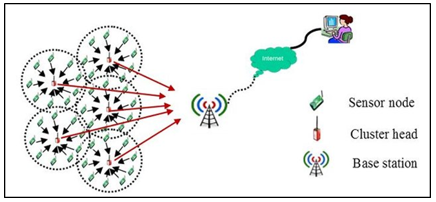Figure 1. A cluster-based WSN model 

2. Review of Literature

This section contains the literature on the PSO and its role in CH selection in WSNs, as well as the research on the early detection of forest fires. The most significant literature regarding the extension of the lifespan of WSNs will be presented first, followed by an explanation of forest fires.

Deepa and Rekha  suggested the PSO method for two problems: clustering and routing. Improved scalability was accomplished via the clustering process, which ensured equal distribution of nodes within clusters, thus avoiding the issue of residual nodes, which drains the energy of sensor nodes and reduces the network's lifespan. However, there is confusion in routing data to the base station.

Preethiya et al.  created the Mobile Double Cluster Head-PSO (MDCH-PSO) method to improve hybrid WSN lifespan and load balancing. It was accomplished by decreasing the amount of energy spent on checking member nodes by CH and the amount of energy spent on mobility management. However, this method suffers from scalability and a lack of a specific sink for the entire network.

Shinde and Bichkar  presented a PSO-based CH selection approach by selecting the most feasible CHs and cluster formation. CH-nodes were chosen based on three parameters: the residual energy of each node, the intra-cluster distance, and the inter-cluster distance. However, they discussed the fitness function extensively in their paper, although it did not exist mathematically.

Sahoo et al.  presented the Energy-Efficient Clustering and Sink Mobility technique based on PSO. The technique was presented to address the issues associated with CH selection and sink mobility, and it combined optimal CH selection with optimal PSO routing. Additionally, the movement of the sink is optimized. Regardless of the outcome, three optimization concerns identified by PSO need significant effort to resolve, especially as the network increases. Additionally, there are five limits in fitness function that need more time to process.

Han et al.  presented the Energy-Balanced Cluster Routing Protocol (EBCRP) as a solution based on (PSO). By this technique, dynamically picking a CH for each cluster in each cycle was done using a rotating CH selection method based on the highest residual energy, but at the cost of network system stability.

Biabani et al.  proposed Energy-Efficient Cluster Head Selection (ECHS) and routing method based hybrid PSO–HSA for CH selection and routing the data by multi-hop to BS. They suggested many objectives in term of energy efficiency, cluster closeness and coverage, and that require more H.W sources.

Loganathan and Arumugam  proposed a new energy-efficient CH-selection method based on PSO to solve disparity clustering and routing issues. They assumed a Super CH between the SN and the CH and sink nodes, chosen by taking the distance parameters between the SN and the CH and sink nodes into account. However, this method was not helpful in the case of homogeneous sensors because it assumed the presence of sensors pre-configured to be super sensors.

After reviewing the aforementioned literature [11-16], it is apparent that. Notwithstanding its success in prolonging the life of WSNs and optimizing CH selection, it is not designed for a particular application. Nonetheless, when applications are added to these techniques, they prove their logic and usefulness.

Noureddine and Bouabdellah  designed a Multimedia Forest Fire System in which cameras would gather information sources. Grover et al.  recommended that the forest be split into square-shaped monitoring clusters, each with its own sensor system. The studies [18, 19] needed a substantial investment in infrastructure and a big number of qualified employees.

To overcome this disadvantage, we assume a PSO-based WSN life extension system for selecting modified CHs via a proper fitness function and deploying the sensors appropriately to achieve the required coverage of events for early detection of forest fires.

3. Particle Swarm Optimization (PSO)

PSO is the simplest metaheuristic optimization approach that is nature-inspired and computationally efficient . The primary goal of PSO is to determine the optimal particle Pi, 1≤i≤Np, location Xi,m that produces the best Pbesti assessment of the fitness function. Each iteration adjusts the velocity Vi,m for particle and, 1≤m≤M, is in the mth dimension of the area of quantification. For all particles, the M dimension is the same of each particle Pi, depending on its current velocity Vi,m, prior local best Pbesti, and global best Gbest position. On the basis of the preceding measurements, the updated velocity Vi,m(t+1) and particle location Xi,m(t+1) may be determined. Each repeat follows a similar method. Eq. (1) and Eq. (2) include mathematical formulae for updating the velocity and location of the particles .

\begin{aligned} V_{i, m}(t+1)=\omega & \times V_{i, m}(t) \\ &+c 1 \times x 1\left(X_{\text {Pbest }}-X_{i, m}\right) \\ &+c 2 \times x 2\left(X_{\text {Gbest }}-X_{i, m}\right) \end{aligned}      (1)

$X_{i, m}(t+1)=X_{i, m}(t)+V_{i, m}(t+1)$      (2)

where, w is inertia weight must be in, 0<w<1, and, 0≤(c1, c2)≤2, are coefficients of acceleration, 0 and 0<(x1, x2)<1 are the values produced randomly . The process is repeated until a suitable value for Gbest is attained. After acquiring a freshly updated position, the Pi analyzes the fitness Pbesti and Gbest for the maximization problem, based on Eqns. (3) and (4).

$P_{\text {besti }}$$=\left\{\begin{array}{lr}P_{i}, & \text { if }\left(\text { Fitness }\left(P_{i}\right)>\text { Fitness }\left(P_{\text {besti }}\right)\right) \\ P_{\text {best } i} & \text { Otherwise }\end{array}\right.$       (3)

$G_{\text {besti }}=\left\{\begin{array}{lr}P_{i}, & \text { if }\left(\text { Fitness }\left(P_{i}\right)>\text { Fitness }\left(G_{\text {best }}\right)\right) \\ G_{\text {best }} & \text { Otherwise }\end{array}\right.$         (4)

4. The Proposed WSN Lifespan Increase Algorithm

4.1 Network model

The WSN scenario described in this research has many characteristics. Clusters of nodes are randomly deployed around the sensing region, with each cluster consisting of six nodes organized hexagonally and staying fixed after deployment. The maximum distance between sensor and event in the field will be d, where d is the distance between two corners, as in Figure 2. Sensors may work as CH or Sensor Node (SN). The sensors are homogenous. Fields of Sensing and communication are circular. Within the transmission range, wireless, symmetrical transmission connections are built between nodes. Nodes send data in different energy levels relying on the distance to the sink.

## 2.png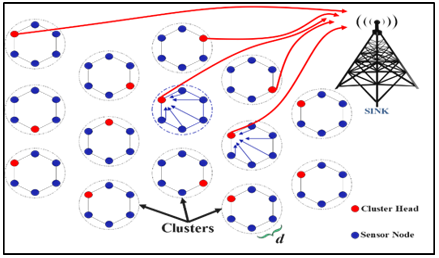Figure 2. Proposed deployment

4.2 Energy model

In our proposal, we use the simple radio model in Ref. . The transmitter requires energy to power the radio and amplifier circuits included inside the wireless sensor node. The quantity of data transferred and the distance travelled dictate the power consumption. Eq. (5) calculates the total energy consumed by each sensor node to Transmit the quantity of bit (l-bit) data.

$E_{T X}(l, d)=\left\{\begin{array}{l}l \times E_{e l e c}+\varepsilon_{f s} \times l \times d^{2} ; \text { if } d<d_{0} \\ l \times E_{e l e c}+\varepsilon_{m p} \times l \times d^{4} ; \text { if } d \geq d_{0}\end{array}\right.$     (5)

where, Eelec is the energy wasted per bit to operate the transmitter or receiver circuit, amplification energy for the free space model εfs and εmp for the multipath model (depending on the transmitter amplifier type), and d0 is the threshold transmission distance. The power consumption of this model depends on the threshold distance d, when the transmission distance is less than $d_{0}$, the power consumption is according to d2, otherwise, is according to d4. The energy consumption to Receive (l-bit) packet of data is in Eq. (6):

$E_{R X}(l)=l \times E_{e l e c}$      (6)

4.3 Proposed algorithm and fitness function

We developed a fitness function that maximizes network longevity. Each cluster's CH node is selected based on its fitness, and it is derived from: Proportional Energy Cluster (PEC); where it is the ratio of the CH's energy to the sum of the cluster's energy SNs, as in Eq. (7):

$\boldsymbol{P} \boldsymbol{E} \boldsymbol{C}=\left[\frac{E_{C H_{j}}}{\sum_{j=1}^{l_{j}} E_{S_{j}}}\right]$     (7)

where, S: A collection of Sensors S={S1, S2, …, Sn}; Cm: NO. of a cluster; lj: NO. of sensors in the cluster j; Rmax: A Node's maximum communication range; TH: The threshold energy for being a cluster head; $E_{C H_{j}}$: The current Energy of the cluster head CHj; $E_{S_{j}}$: The current Energy of the sensor node Sj; dis (CHj, BS): distance between CH and BS; dis (Sj, BS): distance between sensor nodes and BS.

The CHj is selected from sensor nodes Sj inside the cluster for each iteration in PSO. Increasing WSN lifetime done by choice the CH that gives maximum PEC, as in Eq. (8), with three limitations.

$\operatorname{Max} \boldsymbol{P E C}=\frac{E_{\mathrm{CH}_{j}}}{\sum_{j=1}^{l_{j}} E_{S_{j}}}$     (8)

Limitations:

(1) $E_{C H_{j}}>T_{H}$;

(2) $\left\{\operatorname{dis}\left(S_{j}, B S\right)\right.$, and dis $\left.\left(\mathrm{CH}_{j}, B S\right)\right\} \leq R_{\max }$；

(3) $\forall\left(S_{j}\right.$ and $\left.C H_{j}\right) \in C_{m}$;

In the first constraint; The candidate CH-node must have enough power to collect data from its nodes, fuse it, and then transfer it to the BS. In other words, CH has a higher energy than the threshold energy, which is established based on the suggested maximum data amount and maximum distance from the BS.

As a result, our fitness function will be as shown in Eq. (9). The goal is to maximize fitness in Eq. (9). The higher the fitness value, the better the particle location is, and thus the better the selection of the CHs.

$\operatorname{Max} P \boldsymbol{E C}=$ fitness function $(F)$      (9)

where, 0<fitness function (F)<1.

In swarming algorithms, every particle represents a potential solution. Assume that, according to the suggested deployment, each particle Pi(node) has location Xi,m resides at the (xi,m, yi,m) coordinate inside a hexagonal cluster. Locations will be updated according to Eq. (1) and Eq. (2) in each iteration to move to the next location amongst cluster members; Each update, the particle is assessed based on Eq. (3) and Eq. (4), according to the suggested fitness function based on Eq. (9), until only the particle with the global solution remains, that represent the CH. This procedure for all clusters in the search space is done to select the CHs for all clusters. PEC is calculated during each iteration of this node Pi, which is suggested as CH. In other words, this node indicates a possible solution and may be nominated as (CH); the PEC value is computed by dividing the candidate node's remaining energy by the total energy of the cluster's nodes. If the PEC value is high, it will be considered (Pbesti) and saved for comparison with the PEC values of the other nodes in order to determine the ideal CH-node. Consequently, the higher the PEC value, the more often the node with the highest power would be selected to represent as CH, which enhances the WSN's load balance and so increases its lifespan.

The proposed algorithm will be as following steps in Figure 3. The suggested algorithm is intended to achieve two goals: extending the lifetime of WSN and increasing the accuracy of event monitoring. The first goal is achieved by applying the algorithm steps in Figure 3. To achieve the second goal, the sensors are deployed in clusters arranged in a hexagonal form. Much of the literature prefers to deploy nodes in the form of a hexagonal grid because it is characterized by high coverage and high connectivity . However, the deployment of nodes in a continuous hexagonal grid is not easily applied on the ground due to the geographical variety. Continuous hexagonal grid coverage presupposes partitioning the whole search area into hexagonal forms, so generating a hexagonal grid that covers the entire region regardless of the terrain's topography, as in Ref. .

As a result, we proposed deploying the nodes in the shape of discrete hexagonal clusters to provide maximum coverage of the events to be monitored and suit the diverse geographical nature, as in Figure 3.

## 3.png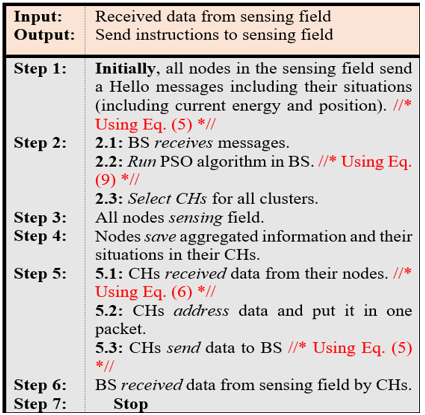Figure 3. Steps of proposed algorithm

5. Case Study and Results

Forest fire disasters in recent times have become a constant problem that occurs every summer in various regions of the world . Early detection of the occurrence of fire contributes to great results in extinguishing it. There are many ways to detect forest fires early; the most famous of these methods is human monitoring, or surveillance, cameras or satellites. However, these technologies suffer from limitations, resulting in delays in the early detection of fires and some being costly .

This paper proposes using a Wireless Sensor Fire Detection (WSFD) system. Sensors will be deployed in high-risk areas to collect data such as smoke detection, temperature, particle change, etc. All the information collected by the sensors will be sent to their CHs as a gateway to transmit data to the BS. As mentioned in the network model section, sensors will be deployed as hexagonal clusters. The proposed PSO selects the CHs for each cluster to extend the network's lifetime. The clusters will be deployed randomly in the forest depending on the natural geography of the forest, as in the Figure 4. The algorithm proposed for early forest fire detection is illustrated in Figure 5.

## 4.png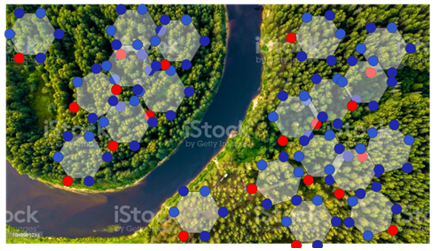Figure 4. Random deployment for clusters as hexagonal shapes in the forest

The operation of the WSFD network for early forest fire detection has been simulated using the MATLAB program version (8-R2012-b). To create a simulation as close as possible to reality, and as a preliminary experiment, we proposed a system for detecting forest fires in a hypothetical forest with an area of (40 km2) that has dimensions (200 m × 200 m). Parameters for each cluster are illustrated in Table 1.

Each cluster covers an area of (800 m2) and has a diameter of (32 m). The sensors are installed on the forest ground, and all sensors are (16 m) away from the cluster's center.

Table 1. Cluster parameters

 Parameter Value Cluster Area 800 m2 Cluster diameter 32 m Distant SN from the center of Cluster 16 m No. of WSFD in Cluster 6

In simulation experiments, the packet length and other network parameters used are defined in Table 2. The information will continue to be broadcast at the last node in the forest stops transmitting, meaning all nodes' power has been spent and all nodes have died.

Table 2. Network parameters

 Parameter Value Area of interest 200 m * 200 m Location of BS (210, 210) and (100, 100) No. of Clusters 20 No. of CH 1 No. of SNs for each Cluster 6 Total Sensors 80 and 120 SN-Energy 2 J d_max 100 m The threshold distance (d0) 30 m Eelec 50 nJ/bit $\boldsymbol{\varepsilon} f s$ 10 pJ/bit/m2 $\varepsilon m p$ 0.0013 pJ/bit/m4 Message size 60000 bit

For CHs-selection, parameters of the proposed PSO in Table 3. The high inertia weight $. \omega$ is conducive to exploration, while the low $. \omega$  is favorable to exploitation. Thus, the weight of inertia influences the prior velocity. Therefore, $. \omega$ was set equal to (0.7) to provide a suitable balance between exploration and exploitation for select CHs nodes.

Table 3. PSO parameters

 Parameter Value No. of Population 30 $\mathcal{C 1}$ 2 $\mathcal{C 2}$ 2 1. $\omega$ 0.7 No. of iterations 20

Results of the simulation in 3-metrics, (1) Alive clusters, (2) Alive sensors, and (3) Remained energy. Where, the Alive Clusters: No. of clusters that have one WSFD or more to monitor the forest in all rounds; Alive Sensors: No. of WSFDs that be alive in all rounds for all sensors in the forest; Remained Energy: Total energy for all nodes in the forest that be consumed during the run the simulation.

Results of the proposed PSO to select CH for each cluster are compared with Random Selection (RS) , LEACH  and Static-CH . The CH will be selected randomly for each round in the RS method. LEACH identifies the CH as in  for each round. The Static-CH defines just one CH for all rounds.

## 5.png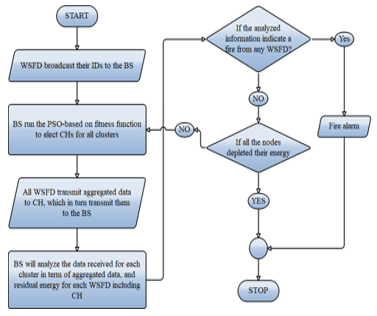Figure 5. Flowchart for the proposed work of the surveillance system for WSFD

5.1 Experiment one

WSFDs are deployed in a hexagonal shape as clusters, as shown in Figure 6. BS placed outfield at (210, 210). Simulations were run to acquire results about the number of live clusters, live WSFDs, and residual energy, as shown in Figure 7, Figure 8, and Figure 9.

## 6.png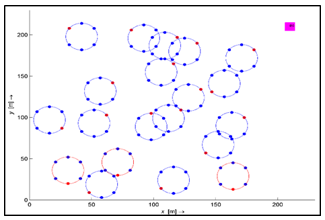Figure 6. Random deployment for hexagonal clusters in the forest

## 7.png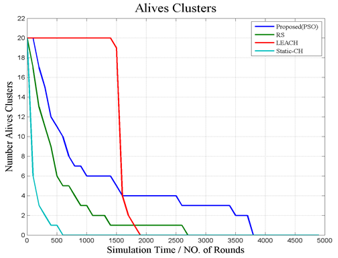Figure 7. Alive clusters in EXP.1

## 8.png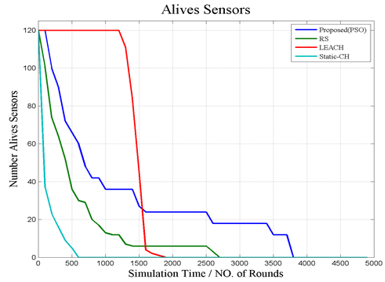Figure 8. Alive WSFDs in EXP. 1

## 9.png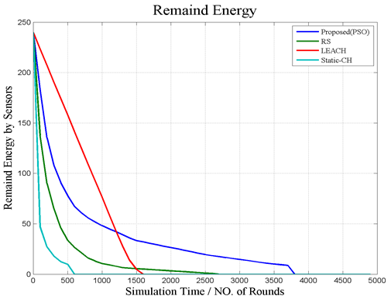Figure 9. Remained energy in EXP. 1

5.2 Experiment two

In this experiment, the same parameters were used in the first experiment, but the location of the BS was assumed in the center of the research area in (100, 100), as shown in Figure 10. The purpose of that is to compare the performance and lifespan of the WSN in the event that the base station is placed to the center of the forest. Simulations were run to acquire results about the number of live clusters, live WSFDs, and residual energy as shown in Figures 11-14.

## 10.png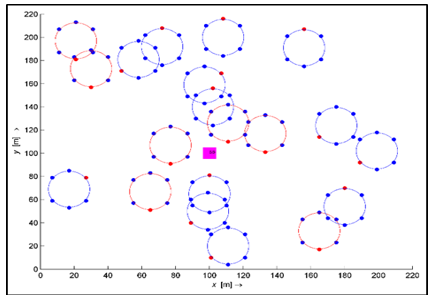Figure 10. Random deployment for hexagonal clusters in the forest

## 12.png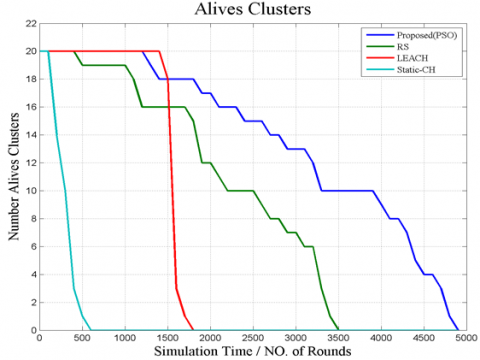Figure 11. Alive clusters in EXP. 2

## 13.png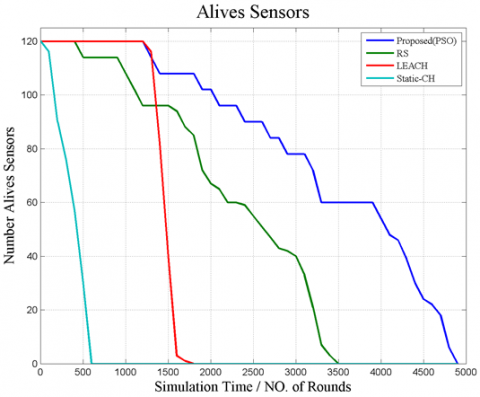Figure 12. Alive WSFDs in EXP. 2

## 14.pngFigure 13. Remained energy in EXP. 2

It is evident in the experiments that selecting one node as static CH results in a significant decrease in the Static-CH method; once the CH node dies, the link to the other nodes is severed, and the cluster dies, even when the remaining related nodes have adequate energy. Although the LEACH protocol achieves good results in terms of extends the life of the network in comparison to static protocols, but subsidence happens in LEACH due to selecting a low-energy CH node, thus eliminating the cluster. In RS, the CH node is chosen randomly or periodically, which cannot be anticipated, resulting in the selection of a low-energy node and, ultimately, the cluster's death.

We remark that the proposed PSO algorithm is preferable because it selects CH based on the best feasible outcome as determined by the proposed fitness function, which produces optimum or near-optimal outputs. Additionally, when the BS is in the center of the field in EXP.2, the proposed PSO achieves superior outcomes in terms of nodes stability and clusters preservation.

The results of the experiments (EXP.1 and EXP.2) indicate that the system is still stable despite the BS location is changing in experiments.

The proposed PSO algorithm is better in terms of extending the life of the network when comparing the results of the experiments using three measures (FSD, HSD, and LSD), as shown in Table 4, and represented by the holographic in Figure 15; where these measures are defined as follows:

(1) First Sensor Dead (FSD): No. of rounds until the first sensor dies.

(2) Half Sensors Dead (HSD): No. of rounds until half of the sensors die.

(3) Last Sensor Dead (LSD): No. of rounds until the last sensor dies.

Table 4. Performance comparison of the experiments

 No. BS Algorithm FSD HSD LSD EX.1 BS at (210, 210) PSO 118 452 4672 RS 112 301 3126 LEACH 1244 1450 1709 ST-CH 23 139 696 EX.2 BS at (100, 100) PSO 1281 3968 4839 RS 516 2075 3514 LEACH 1205 1437 1661 ST-CH 292 631 800

## 15.png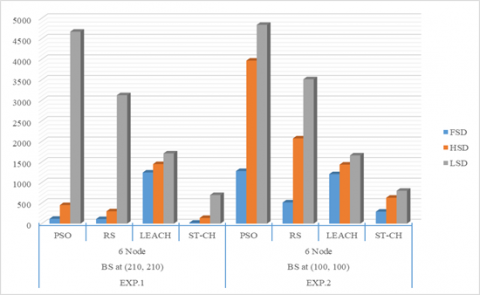Figure 14. Lifetime of Sensors in two experiments

6. Conclusions

Several conclusions were reached in this paper; the most important is that choosing the CH node is the effective way to increase the WSN lifetime. Among the experimental methods, PSO is the most dependable. The optimal deployment of sensors as clusters provides high flexibility in event coverage and ensures a balanced distribution of energy consumption for WSN. The proposed fitness function based on the PSO used to determine the optimal CH based on residual energy produced good results in extending the network's lifespan. The application of WSFD work in the early detection of forest fires has been simulated by using the proposed system in this simulation, which ensures high and realistic coverage of the geographical nature of forests. As a result, early detection produces excellent results in terms of environmental preservation and saves significant effort in extinguishing forest fires. The suggested PSO-based algorithm produced favorable outcomes in terms of WSN lifetime, stability, low cost, and few qualified personnel.

Acknowledgment

The authors would like to thank the Control and Systems Engineering Department at the University of Technology and the South Refineries Company (SRC) of the Iraqi Oil Ministry for their support with this work.

References

 Naser, S.M., Croock, M.S. (2018). Proposed simulator based on developed lightweight authentication and key management protocol for wireless sensor network. International Journal of Computing and Digital Systems, 7(4): 251-260. http://dx.doi.org/10.12785/ijcds/070409

 Mutar, H.I., Jawad, M.M. (2022). Enhancing energy utilization in wireless sensor networks (WSNs) by particle swarm optimization (PSO). Webology, 19(1): 6089-6107. https://www.webology.org/abstract.php?id=1337.

 Hashim, S.A., Jawad, M.M., Wheedd, B. (2020). Study of energy management in wireless visual sensor networks. Iraqi Journal of Computers, Communications, Control and Systems Engineering, 20(1): 68-75. http://dx.doi.org/10.33103/uot.ijccce.20.1.7

 Hashim, S.A., Jawad, M.M., Wheedd, B. (2020). A sustainable scheme for minimizing energy in visual sensor network using disjoint set cover approach. Engineering and Technology Journal, 38(12): 1818-1831. http://dx.doi.org/10.30684/etj.v38i12A.1561

 Boyinbode, O., Le, H., Mbogho, A., Takizawa, M., Poliah, R. (2010). A survey on clustering algorithms for wireless sensor networks. In 2010 13th International Conference on Network-Based Information Systems, pp. 358-364. http://dx.doi.org/10.1109/NBiS.2010.59

 Rafea, S.A., Kadhim, A.A. (2019). Routing with energy threshold for WSN-IoT based on RPL protocol. Iraqi J. Comput. Commun. Control Syst. Eng, 19: 71-81. http://dx.doi.org/10.33103/uot.ijccce.19.1.9

 Rao, P.C., Jana, P.K., Banka, H. (2017). A particle swarm optimization based energy efficient cluster head selection algorithm for wireless sensor networks. Wireless Networks, 23(7): 2005-2020. http://dx.doi.org/10.1007/s11276-016-1270-7

 Latiff, N.A., Tsimenidis, C.C., Sharif, B.S. (2007). Energy-aware clustering for wireless sensor networks using particle swarm optimization. In 2007 IEEE 18th International Symposium on Personal, Indoor and Mobile Radio Communications, pp. 1-5. http://dx.doi.org/10.1109/PIMRC.2007.4394521

 Abdulkareem, A.I., Abd Dhahad, H., Yousif, N.Q. (2018). Prospect theory in particle swarm optimization for constraints nonlinear optimization problems. In 2018 Third Scientific Conference of Electrical Engineering (SCEE), pp. 144-149. http://dx.doi.org/10.1109/SCEE.2018.8684144

 Kulkarni, R.V., Venayagamoorthy, G.K. (2010). Particle swarm optimization in wireless-sensor networks: A brief survey. IEEE Transactions on Systems, Man, and Cybernetics, Part C (Applications and Reviews), 41(2): 262-267. http://dx.doi.org/10.1109/TSMCC.2010.2054080

 Deepa, S.R., Rekha, D. (2018). Cluster optimization in Wireless sensor networks using particle swarm optimization. In Annual Convention of the Computer Society of India, pp. 240-253. http://dx.doi.org/10.1007/978-981-13-1343-1_23

 Preethiya, T., Muthukumar, A., Durairaj, S. (2020). Double cluster head heterogeneous clustering for optimization in hybrid wireless sensor network. Wireless Personal Communications, 110(4): 1751-1768. http://dx.doi.org/10.1007/s11277-019-06810-3

 Shinde, A.S., Bichkar, R.S. (2020). Optimal cluster head selection and clustering for WSN using PSO. Int. J. Innov. Technol. Explor. Eng., 9(3): 389-395. http://dx.doi.org/10.35940/ijitee.B7607.019320

 Sahoo, B.M., Amgoth, T., Pandey, H.M. (2020). Particle swarm optimization based energy efficient clustering and sink mobility in heterogeneous wireless sensor network. Ad Hoc Networks, 106: 102237. http://dx.doi.org/10.1016/j.adhoc.2020.102237

 Han, Y., Byun, H., Zhang, L. (2020). Energy-balanced cluster-routing protocol based on particle swarm optimization with five mutation operators for wireless sensor networks. Sensors, 20(24): 7217. http://dx.doi.org/10.3390/s20247217

 Biabani, M., Fotouhi, H., Yazdani, N. (2020). An energy-efficient evolutionary clustering technique for disaster management in IoT networks. Sensors, 20(9): 2647. http://dx.doi.org/10.3390/s20092647

 Loganathan, S., Arumugam, J. (2021). Energy efficient clustering algorithm based on particle swarm optimization technique for wireless sensor networks. Wireless Personal Communications, 119(1): 815-843. http://dx.doi.org/10.1007/s11277-021-08239-z

 Noureddine, H., Bouabdellah, K. (2020). Field experiment testbed for forest fire detection using wireless multimedia sensor network. International Journal of Sensors Wireless Communications and Control, 10(1): 3-14. http://dx.doi.org/10.2174/2210327909666190219120432

 Grover, K., Kahali, D., Verma, S., Subramanian, B. (2020). WSN-based system for forest fire detection and mitigation. In Emerging technologies for agriculture and environment, pp. 249-260. http://dx.doi.org/10.1007/978-981-13-7968-0_19

 Rao, P.C., Jana, P.K., Banka, H. (2017). A particle swarm optimization based energy efficient cluster head selection algorithm for wireless sensor networks. Wireless Networks, 23(7): 2005-2020. http://dx.doi.org/10.1007/s11276-016-1270-7

 Raheem, F.A., Flayyih, M.K. (2017). Quadruped robot creeping gait stability analysis and optimization using PSO. In 2017 Second Al-Sadiq International Conference on Multidisciplinary in IT and Communication Science and Applications (AIC-MITCSA), pp. 79-84. http://dx.doi.org/10.1109/AIC-MITCSA.2017.8722979

 Heinzelman, W.R., Chandrakasan, A., Balakrishnan, H. (2000). Energy-efficient communication protocol for wireless microsensor networks. In Proceedings of the 33rd Annual Hawaii International Conference on System Sciences, p. 10. http://dx.doi.org/10.1109/HICSS.2000.926982

 Priyadarshi, R., Gupta, B., Anurag, A. (2020). Deployment techniques in wireless sensor networks: A survey, classification, challenges, and future research issues. The Journal of Supercomputing, 76(9): 7333-7373. http://dx.doi.org/10.1007/s11227-020-03166-5

 Aslan, Y.E., Korpeoglu, I., Ulusoy, Ö. (2012). A framework for use of wireless sensor networks in forest fire detection and monitoring. Computers, Environment and Urban Systems, 36(6): 614-625. http://dx.doi.org/10.1016/j.compenvurbsys.2012.03.002

 Al Arabiya NEWS. The world is burning. Learn about the list of burning countries. Al Arabiya NEWS website, 2021. https://ara.tv/mukcs.

 Kadir, E. A., Irie, H., Rosa, S.L. (2019). Modeling of wireless sensor networks for detection land and forest fire hotspot. In 2019 International Conference on Electronics, Information, and Communication (ICEIC), pp. 1-5. http://dx.doi.org/10.12928/telkomnika.v17i6.12971

 Chen, J.S., Hong, Z.W., Wang, N.C., Jhuang, S.H. (2010). Efficient cluster head selection methods for wireless sensor networks. Journal of Networks, 5(8): 964. http://dx.doi.org/10.4304/jnw.5.8.964-970

 Huang, B., Hao, F., Zhu, H., Tanabe, Y., Baba, T. (2006). Low-energy static clustering scheme for wireless sensor network. In 2006 International Conference on Wireless Communications, Networking and Mobile Computing, pp. 1-4. http://dx.doi.org/10.1109/WiCOM.2006.250